WASM支持将C/C++/Go/Rust等语言实现的代码编译为浏览器可执行的机器码，从而支持浏览器以接近原生应用的性能运行相关应用。在边缘计算领域，WASM也在尝试建立自己的用例，为边缘应用提供统一的运行环境。原文：Introduction to WebAssembly (WASM)JavaScript有什么问题？

WebAssembly是如何工作的？

WASM是Web应用的未来吗？

# JavaScript有什么问题？Javascript不是为高性能而开发的

JavaScript是由Brenden Eich在1995年为Netscape浏览器设计的，在那个年代，它可以为静态网页实现一些交互。

JavaScript是解释型语言，也是动态类型语言。如果变量类型是在运行时决定的，那么就是动态类型语言。那么动态类型语言的问题是什么呢？

``int x = 5 ;``Javascript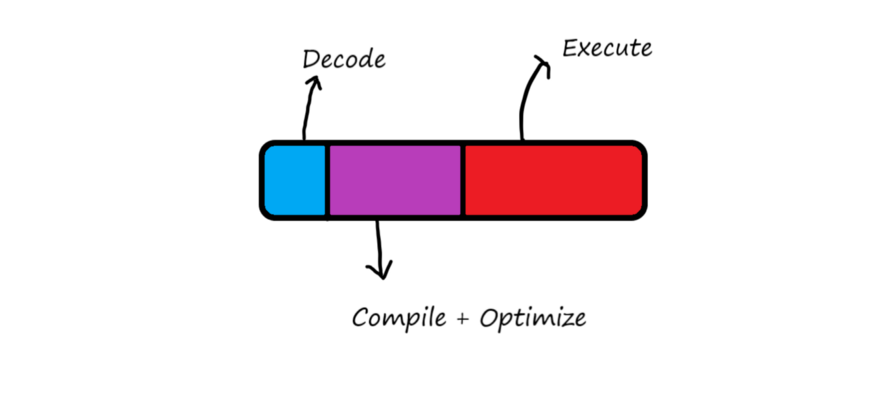WebAssembly

JavaScript不是为CPU密集型和高性能应用程序开发的。

# 什么是WebAssembly？

VirtualProcessor原理图，它将C++代码转换为浏览器可理解的二进制代码。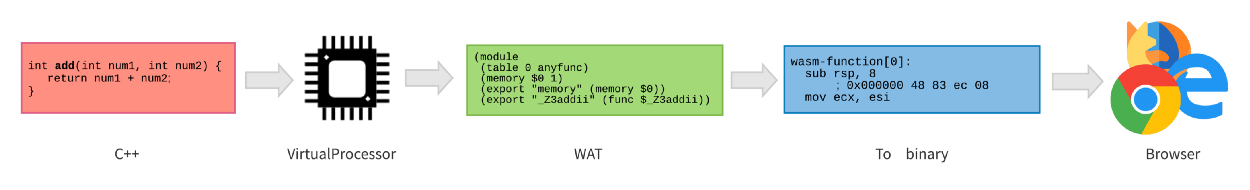# 这是一种类似于C/C++那样的新的编程语言吗？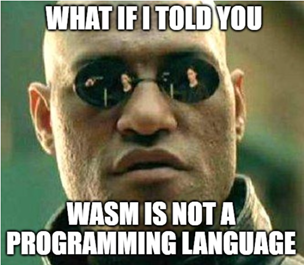确切地说，WASM不是一种编程语言

WASM不是一种编程语言🙂。简而言之，它是一种将用一种编程语言编写的代码转换为浏览器可理解的机器代码的技术。

WASM (WebAssembly的缩写)被设计为其他语言的编译目标，允许服务器端代码(如C或C++代码)被编译成WASM并在浏览器中执行。# WebAssembly是如何工作的？

• 每个处理器都有一个类似x86或ARM的架构。此外，处理器只能理解机器码。
• 编写机器码是件乏味的事情，所以我们用汇编语言来编写。
• 汇编程序将汇编语言中的指令转换为处理器能理解的机器码。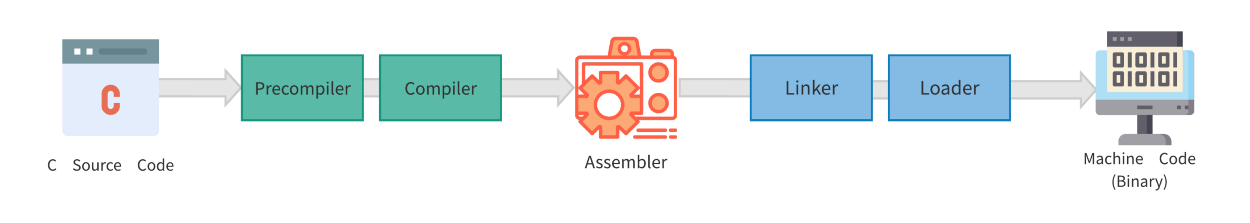# WebAssembly入门

WebAssembly是一个具有WASM扩展名的文件，可以把它看作一个可以导入JavaScript程序的模块。

### 项目目录中的交互文件 —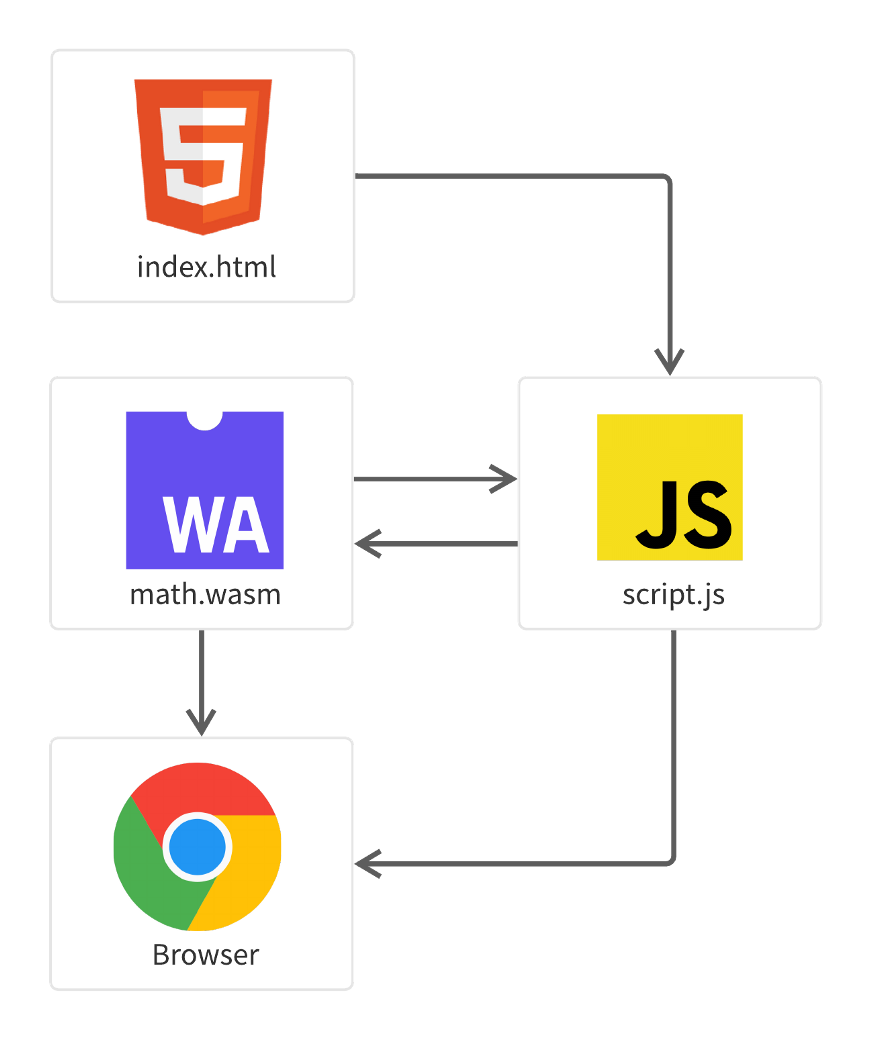#### 1. 用你喜欢的语言编写应用程序。

``````// Following is a function that finds nth fibonacci written in C++
int fib(int n)
{
if (n <= 1)
return n;
return fib(n-1) + fib(n-2);
}``````

#### 2. 创建WASM模块。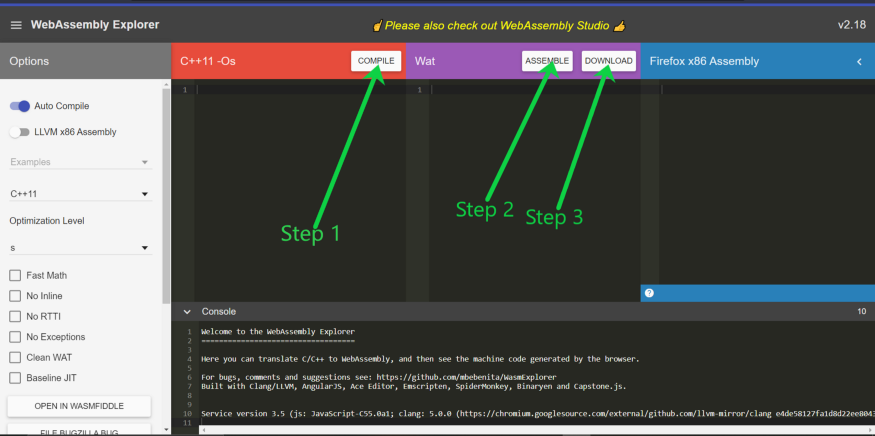编译和汇编之后(步骤1，步骤2)

#### 3. 分发模块——通常可以使用CDN实现低延迟分发，但在这个演示中，我们只在本地运行WASM文件。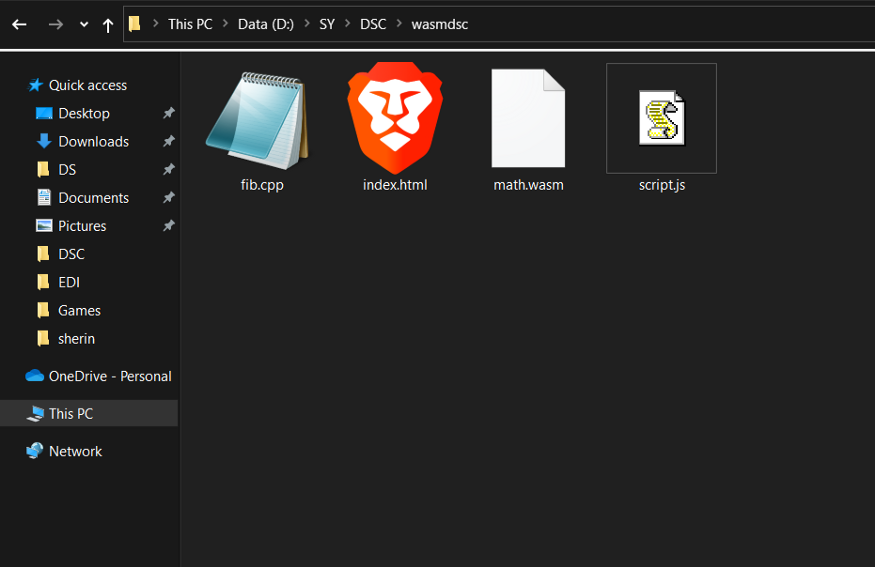项目目录视图

#### 4. 加载WASM模块

``````let math;
// Let's create a function called loadWebAssembly that converts given file into binary array buffer.
return fetch(fileName)
.then(response => response.arrayBuffer())
.then(buffer => WebAssembly.compile(buffer)) // Buffer converted to Web Assembly
.then(module => {return new WebAssembly.Instance(module) }); // Instance of Web assmebly module is returened
};

//We call the function for math.wasm for the given Instance.
.then(instance => {

}); ``````

#### 5. 创建模块实例。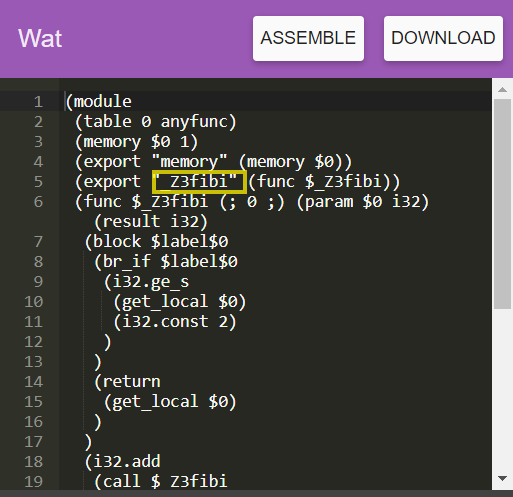要引用的变量加了下划线

`script.js`中，PART 1  是关于加载WASM文件。

PART 2 提供了一些简单的javascript函数，用于比较javascript和WebAssembly的性能。🤓

``````//---------------------------PART 1--------------------------------------------------------
// Let's create a function called loadWebAssembly that converts given file into binary array buffer.
return fetch(fileName)
.then(response => response.arrayBuffer())
.then(buffer => WebAssembly.compile(buffer))
.then(module => {return new WebAssembly.Instance(module) });
};

//We call the function for math.wasm
.then(instance => {
fibc = instance.exports._Z3fibi;

});

//---------------------------PART 2 -----------------------------------------------
// Function written in Javascript for nth fibonacci
function fibj(n)
{
if (n <= 1)
return n;
return fibj(n-1) + fibj(n-2);
}

//This function gives the time required for C++ function
function perfoc(n){
var startTime = performance.now()

var c=fibc(n)

var endTime = performance.now()

console.log(`Calculating nth Fibonacci with WASM took \${endTime - startTime} milliseconds,nth fibonacci is \${c}`)

}

// This function gives the time required for Javascript function
function perfoj(n){
var startTime = performance.now()

var j=fibj(n)

var endTime = performance.now()

console.log(`Calculating nth Fibonacci with JS took \${endTime - startTime} milliseconds, nth fibonacci is \${j}`)

}``````

#### 6. 调用实例函数。

1. `fibj()`→这是用简单的Javascript编写的。
2. `fibc()`→这是用C++编写的，然后转换为WebAssembly。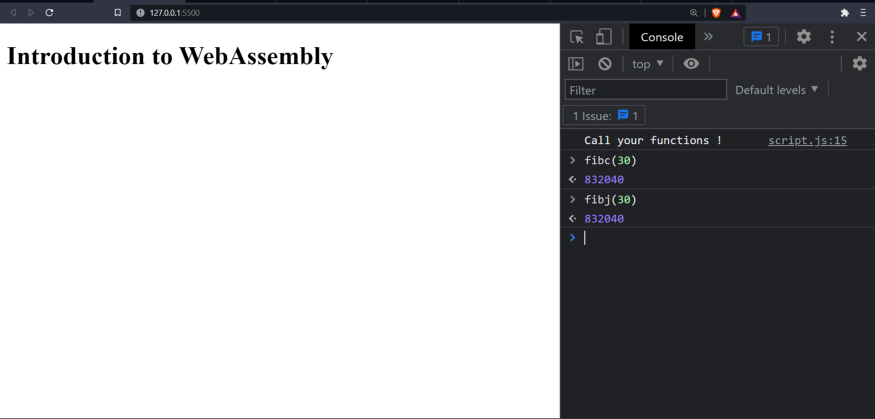两个函数，fibj()和fibc()WebAssembly快得多

`fibj()``fibc()`的执行时间是通过以下函数度量的：

1. `perfoj()`→告诉我们`fibj()`所消耗的时间
2. `perfoc()`→告诉我们`fibc()`所消耗的时间

# WebAssembly是Web应用的未来吗？

：官方网站。什么是WebAssembly？https://webassembly.org/